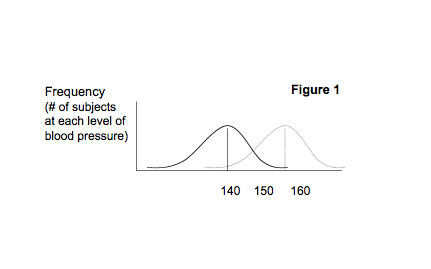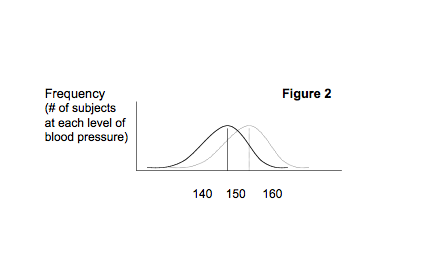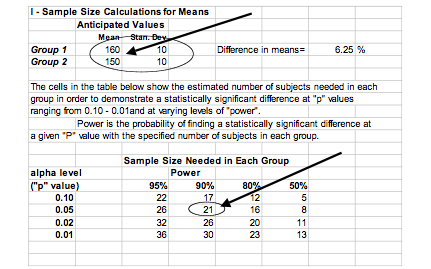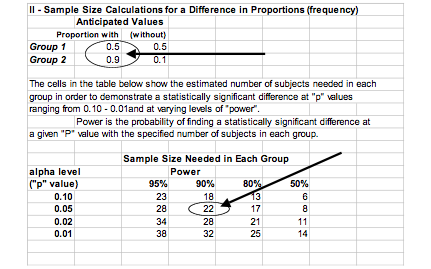BU IACUCInstitutional Animal Care and Use Committee IACUC oversee... Revised March 2021, Approved April 2021

## Purpose

Boston University (BU) is committed to observing Federal policies and regulations and the Association for Assessment and Accreditation of Laboratory Animal Care (AAALAC) International standards for the humane care and use of animals.  This policy provides guidance on sample size calculations.

## Covered Parties

This policy covers all animals on BU premises used for research, teaching, training, breeding, and related activities, hereinafter referred to collectively as “activities”, and is applicable to all persons responsible for conducting activities involving live vertebrate animals at or under the auspices of Boston University.

## University Policy

### Sample-Size Calculations

Estimation of the number of subjects required to answer an experimental question is an important step in planning a study. On one hand, an excessive sample size can result in waste of animal life and other resources, including time and money, because equally valid information could have been gleaned from a smaller number of subjects. However, underestimates of sample size are also wasteful, since an insufficient sample size has a low probability of detecting a statistically significant difference between groups, even if a difference really exists. Consequently, an investigator might wrongly conclude that groups do not differ, when in fact they do.

### What Is Involved In Sample Size Calculations

While the need to arrive at appropriate estimates of sample size is clear, many scientists are unfamiliar with the factors that influence determination of sample size and with the techniques for calculating estimated sample size. A quick look at how most textbooks of statistics treat this subject indicates why many investigators regard sample-size calculations with fear and confusion.

While sample-size calculations can become extremely complicated, it is important to emphasize, first, that all of these techniques produce estimates, and, second, that there are just a few major factors influencing these estimates. As a result, it is possible to obtain very reasonable estimates from some relatively simple formulae.

When comparing two groups, the major factors that influence sample size are:

1. How large a difference you need to be able to detect.
2. How much variability there is in the factor of interest.
3. What “p” value you plan to use as a criterion for statistical “significance.”
4. How confident you want to be that you will detect a “statistically significant” difference, assuming that a difference does exist.

### An Intuitive Look at a Simple Example

Suppose you are studying subjects with renal hypertension, and you want to test the effectiveness of a drug that is said to reduce blood pressure. You plan to compare systolic blood pressure in two groups, one which is treated with a placebo injection, and a second group which is treated with the drug being tested. While you don’t yet know what the blood pressures will be in each of these groups, just suppose that if you were to test a ridiculously large number of subjects (say 100,000) treated with either placebo or drug, their systolic blood pressures would follow two clearly distinct frequency distributions, as shown in Figure 1.As you would expect, both groups show some variability in blood pressure, and the frequency distribution of observed pressures conforms to a bell shaped curve. As shown here, the two groups overlap, but they are clearly different; systolic pressures in the treated group are an average of 20 mm Hg less than in the untreated controls.

Since there were 100,000 in each group, we can be confident that the groups differ. Now suppose that although we treated 100,000 of each, we only obtained pressure measurements from only three in each group, because the pressure measuring apparatus broke. In other words we have a random sample of N=3 from each group, and their systolic pressures are as follows:

 Placebo Group Treated Group 160 155 150 140 140 140

Pressures are lower in the treated group, but we cannot be confident that the treatment was successful. There is a distinct possibility that the difference we see is just due to chance, since we took a small random sample. So the question is: how many would we have to measure (sample) in each group to be confident that any observed differences were not simply the result of chance?

How large a sample is needed depends on the four factors listed above. To illustrate this intuitively, suppose that the blood pressures in the treated and untreated subjects were distributed as shown in Figure 2 or in Figure 3.In Figure 2 the amount of variability is the same, but the difference between the groups is smaller. It makes sense that you will need a larger sample to be confident that differences in your sample are real.In Figure 3 the difference in pressures is about the same as it was in Figure 1, but there is less variability in pressure readings within each group. Here it seems obvious that a smaller sample would be required to confidently determine a difference.

The size of the sample you need also depends on the “p value” that you use. A “p value” of less than 0.05 is frequently used as the criterion for deciding whether observed differences are likely to be due to chance. If p<0.05, it means that the probability that the difference you observed was due to chance is less than 5%. If want to use a more rigid criterion (say, p<0.01) you will need a larger sample. Finally, the size of the sample you will need also depends on “power,” that is the probability that you will observe a statistically significant difference, assuming that a difference really exists.

To summarize, in order to calculate a sample-size estimate if you need some estimate of how different the groups might be or how large a difference you need to be able to detect, and you also need an estimate of how much variability there will be within groups. In addition, your calculations must also take in account what you want to use as a “p value” and how much “power” you want.

### The Information You Need To Do Sample Size Calculations

Since you haven’t actually done the experiment yet, you won’t know how different the groups will be or what the variability (as measured by the standard deviation) will be. But you can usually make reasonable guesses. Perhaps from your experience (or from previously published information) you anticipate that the untreated hypertensive subjects will have a mean systolic blood pressure of about 160 mm Hg with a standard deviation of about +10 mm Hg. You decide that a reduction in systolic blood pressure to a mean of 150 mm Hg would represent a clinically meaningful reduction. Since no one has ever done this experiment before, you don’t know how much variability there will be in response, so you will have to assume that the standard deviation for the test group is at least as large as that in the untreated controls. From these estimates you can calculate an estimate of the sample size you need in each group.

### Sample Size Calculations For A Difference In Means

The actual calculations can get a little bit cumbersome, and most people don’t even want to see equations. Consequently, I have put together a spreadsheet (Lamorte’s Power Calculations) which does all the calculations automatically. All you have to do is enter the estimated means and standard deviations for each group. In the example shown here, I assumed that my control group (group 1) would have a mean of 160 and a standard deviation of 10. I wanted to know how many subjects I would need in each group to detect a significant difference of 10 mm Hg. So, I plugged in a mean of 150 for group 2 and assumed that the standard deviation for this group would be the same as for group 1.The spreadsheet actually generates a table which shows estimated sample sizes for different “p values” and different power levels. Many people arbitrarily use p=0.05 and a power level of 80%. With these parameters you would need about 16 subjects in each group. If you want 90% power, you would need about 21 subjects in each group.

The format in this spreadsheet makes it easy to play “what if.” If you want to get a feel for how many subjects you might need if the treatment reduces pressures by 20 mm Hg, just change the mean for group 2 to 140, and all the calculations will automatically be redone for you.

### Sample Size Calculations For A Difference In Proportions

The bottom part of the same spreadsheet generates sample-size calculations for comparing differences in frequency of an event. Suppose, for example, that a given treatment was successful 50% of the time and you wanted to test a new treatment with the hope that it would be successful 90% of the time. All you have to do is plug these (as fractions) into the spreadsheet, and the estimated sample sizes will be calculated automatically as shown here:

The illustration from the spreadsheet below shows that to have a 90% probability of showing a statistically significant difference (using p< 0.05) in proportions this great, you would need about 22 subjects in each group.The Statistical Explanation Sample Spreadsheet described above can be found here.

### Responsible Parties

Principal Investigators are responsible for: preparing and submitting applications; making modifications in applications in order secure IACUC approval; ensuring adherence to approved protocols, and ensuring humane care and use of animals. It is the responsibility of the IACUC to assure that the number of animals to be used in an animal use protocol is appropriate. The Animal Welfare Program and the Institutional Animal Care and Use Committee are responsible for overseeing implementation of and ensuring compliance with this policy.

## History

Effective Date:            04/06/2021

Next Review Date:      04/05/2024

First Approved:           02/2012

Revised:                         01/2014, 05/2018